GRE Subject Test: Chemistry : Variance and Standard Deviation

Example Questions

Example Question #1 : How To Find Standard Deviation Of A Random Variable

Robert's work schedule for next week will be released today.  Robert will work either 45, 40, 25, or 12 hours.  The probabilities for each possibility are listed below:

45 hours: 0.3

40 hours: 0.2

25 hours: 0.4

12 hours: 0.1

What is the standard deviation of the possible outcomes?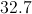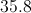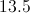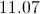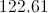Explanation:

There are four steps to finding the standard deviation of random variables. First, calculate the mean of the random variables. Second, for each value in the group (45, 40, 25, and 12), subtract the mean from each and multiply the result by the probability of that outcome occurring. Third, add the four results together. Fourth, find the square root of the result.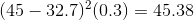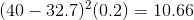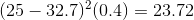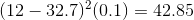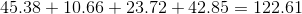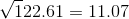Example Question #1 : How To Find Standard Deviation Of A Random Variable

We have two independent, normally distributed random variables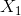and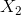such thathas meanand varianceandhas meanand variance. What is the probability distribution of the difference of the random variables,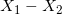?

Normal distribution with meanand variance.

Normal distribution with meanand variance.

Normal distribution with meanand variance.

Normal distribution with meanand variance.

Normal distribution with meanand variance.

Explanation:

The mean for any set of random variables is additive in the sense that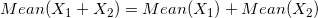The difference is also additive, so we have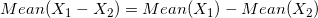This means the mean ofisThe variance is additive when the random variables are independent, which they are in this case. But it's additive in the sense that for any real numbers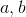(even when negative), we have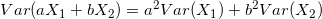.

So for this difference, we have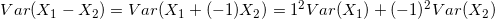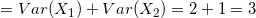.

So the mean and variance areand, respectively. In addition to that,is normally distributed because the sum or difference of any set of independent normal random variables is also normally distributed.

All GRE Subject Test: Chemistry Resources